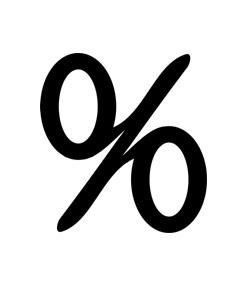# Shortcuts - Percentage Conversions

6 Questions | Attempts: 832
ShareSettingsThis is designed for Wicomico county ALC.   The accompanying lesson can be found on https://wicomicoadultlearning. Wikispaces. Com/Conversions+to+percentages

• 1.
What is 1% of 13?
• A.

1.3

• B.

.013

• C.

.13

• D.

13

• 2.
What is 1000% of 53.5?
• A.

53.5

• B.

107.0

• C.

5.35

• D.

535.0

• 3.
What is 20% of 200?
• A.

40

• B.

20

• C.

4

• D.

2

• 4.
10% of 5000 is 50.
• A.

True

• B.

False

• 5.
10% of 5000 is 500
• A.

True

• B.

False

• 6.
20% of 5000 = 10% of 5000 + 10% of 5000 = 2 x (10% of 5000)
• A.

True

• B.

False

## Related TopicsBack to top
×

Wait!
Here's an interesting quiz for you.